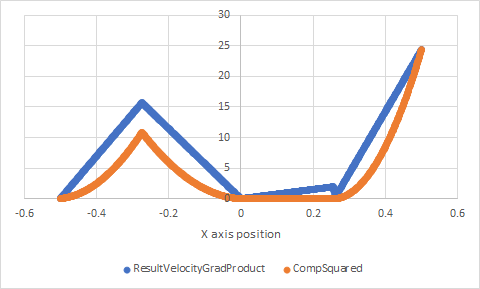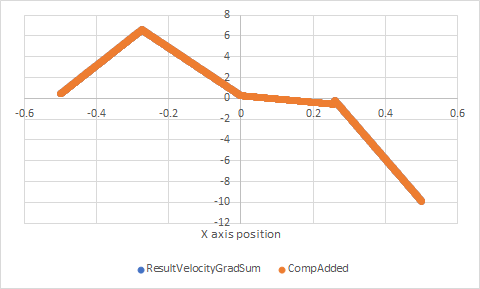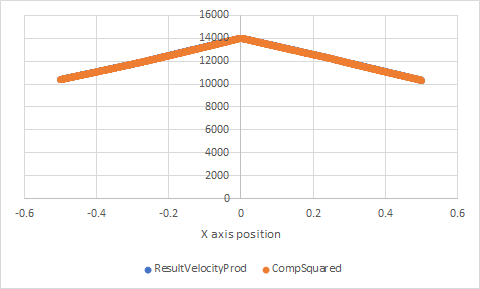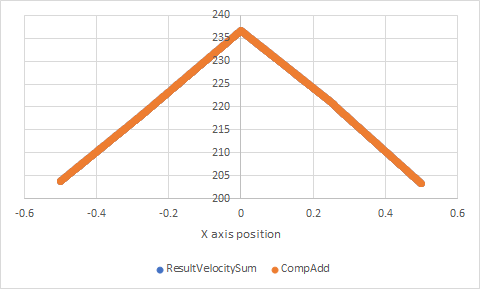# Unexpected multiplication result in Calculator filter using gradient components

I have been having some trouble with the Calculator filter in a very specific case involving the components of the Gradient of Unstructured Data Set filter. Attached are two files which have been able to demonstrate this issue.

myblock.vtk (168.6 KB) testcase.pvsm (576.9 KB)

The testcase.pvsm file opens the myblock.vtk file which contains a scalar field and a vector field. Running the components of the vector field through the gradient filter, I then attempt to multiply two components of the resulting vector of gradients, for example “thexversion_1*thexversion_1”. In the example case, the probe shows that “thexversion_1” has the value 0.124328, and so I would expect the product to yield ~0.155, but the probe shows that the result of the product is ~0.049. If I add the components I do get the correct result, it seems to be only multiplication that is incorrect. Trying this with other fields, such as the vector components or position components, seems to work just fine with addition and multiplication, so it appears to be specific to the results of the gradient filter.

This has occurred with Paraview ParaView-5.6.1-Windows-msvc2015-64bit and ParaView-5.7.0-RC2-Windows-Python2.7-msvc2015-64bit on Windows 10 64bit.

Your probe is between points, so there is interpolation of values, which breaks the equality. If you check the individual values in the results of the calculator instead of using the probe, or if you put your probe on an actual mesh point using Ctrl-P, the results are correct.

Hi Mathieu, thank you for your reply. At your suggestion I did use Ctrl-P to center the probe on a mesh point which shows the value of the gradient product is as expected, just as you said, but I am still wondering why this is so far from the correct answer when on the interpolated points between the mesh, and one is probing or plotting over a line, and using the gradient. I had thought that this might be the result of interpolation, but the deviation seems substantially larger specifically for the product when the gradient filter is used, and so I’m concerned that this is more than just interpolation.

Here are the discrepancies I’m seeing for the test file I previously attached when plotting over an axis (The data set that begins with “Result” is the result of the Calculator filter in Paraview taking a component and then multiplying or adding it to itself, and the data set that begins with “Comp” is that same component having the same operation done through Excel) :

Comparison of a component of the gradient of velocity multiplied by itself:Comparison of a component of the gradient of velocity added to itself:Comparison of a component of the velocity multiplied by itself:Comparison of a component of the velocity added to itself:(Here are the state file and the spreadsheet I used to do all the calculations, for posterity:
comparisonstate.pvsm (712.3 KB)
CubePlotComparisons.xlsx (580.1 KB) )

As seen in the pictures, it seems to be only the combination of gradient filter and multiplication in the calculator filter that leads to the large difference, all 3 of the other comparisons overlap well. In another test case where I originally found this problem, the deviation was several orders of magnitude, taking a velocity gradient component that was probed to be 0.0263399, squaring it in the calculator filter, and probing it to get 2.69488 rather than the expected ~0.00069 (and again, this process did give acceptable answers for other procedures, like sums).

If this is still purely due to the interpolation, do you have any suggestions about avoiding this problem when I have to invoke multiplication of the gradient components?

Nice analysis.

The results have nothing to do with Gradients or Vectors but with the presence of negative values in the initial array (Vector_X or the thexversion_1). The interpolation with the negatives values seems to produce results the breaks the equality.